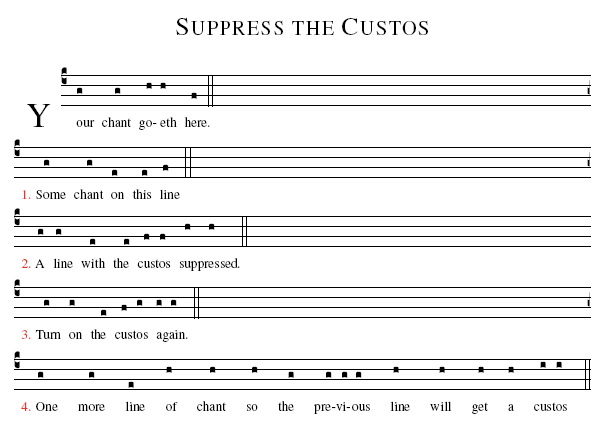#gregowiki

### Site Tools

using_macros_in_your_gabc

# Using Macros in gabc

You can use tex macros right in your gabc scores. Here is why you would want to do that:

• You need a certain effect for just a particular score and you don't want to change your tex template for just one production.
• You need a particular effect on just one part of your score, or maybe just one note. You can't effect this in your tex template.

`def-m1:\yourmacro;`
• A macro will always be some tex code that you want to use in the middle of your gabc score.
• You need to know what tex code you want! (look here and here)
• Sometimes you may need more than one macro so you can enable and disable the tex coding (as in our example below)
• You can have up to 9 macros in a gabc score.
• You define a macro like this:``def-m1:\yourmacro``
• You have to define them in the gabc header with: ``def-m1``, ``def-m2``, ``def-m3``, etc.
• Macros can only be numbered from 1 to 9 (In other words, you can't use ``def-m10``).
• To use a macro in a particular place, you put it inside the parenthesis of the chant notes.
• Also, the coding engine knows it is a macro only because you put it inside double-brackets. That means that you call your macro like this: ``[em1]``. And notice the ``e`` there - that stands for execute, as in execute m1

That all sounds like a lot, but it's not so bad. So, to summarize:

2. Use the prefix to execute the macro: ``em1``
3. Put the macro in brackets: ``[em1]``
4. Then put it inside your chant notes: ``my(g) chant(h[em1])``

### Example 1: Suppress the Custos

For our example, let's pretend you want to suppress the automatic custos for just a single staff, and then allow it again.

You place this in the gabc header.

```name:Suppress the Custos;
def-m1:\greblockcustos;
def-m2:\greblockcusto=0;
%%
(c4)Your(g) chant(g) go(h)eth(h) here.(f) (::)(Z)
<c>1.</c> Some(g) chant(g) on(e) this(e) line(f) (::Z)
<c>2.</c> A(g) line(g) with(e) the(e) cus(f)tos(f) sup(h)pressed.(h) (::Z[em1])
<c>3.</c> Turn(g[em2]) on(g) the(e) cus(f)tos(g) a(g)gain.(g) (::Z)
<c>4.</c> One(g) more(g) line(e) of(h) chant(h) so(h) the(g) pre(g)vi(g)ous(g) line(h) will(h) get(h) a(h) cus(i)tos(i) (::)```And, once you've suppressed your custos, you can manually add it just after the double bars by putting a ``h+`` or ``g+`` after your double-bars (``::``).

### Example 2: Make it Green

In this example, we'll make a section of the chant green! If you just want red, you can surround your gabc notes with ``<c>…</c>``, but if you want green, you'll have to use a macro.

```name:Make It Green;
def-m1:\color{green};
def-m2:\color{black};
%%
(c4)Your(g) chant(g) go(h)eth(h) here.(f) (::)
([em1])This(g) section(g) will(e) be(e) green(f) ([em2]::)
Turn(g) on(g) the(e) black(f) col(g)or(g) a(g)gain.(g) (::)```Back to Start Page